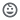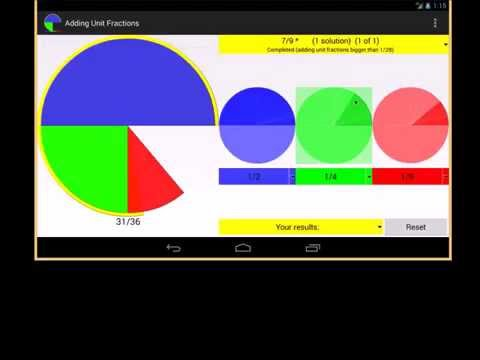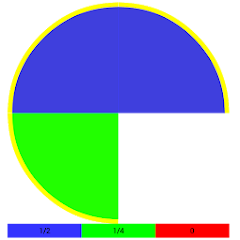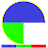10K+Everyone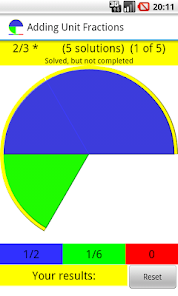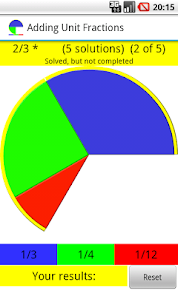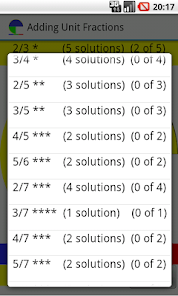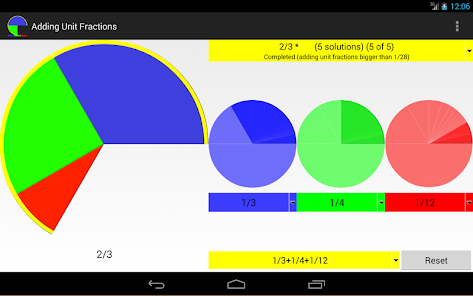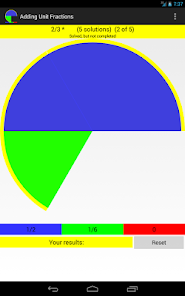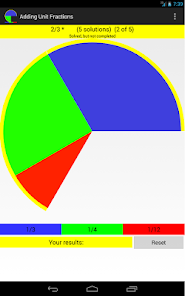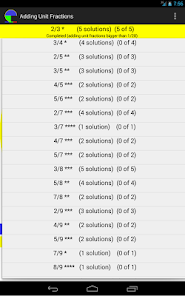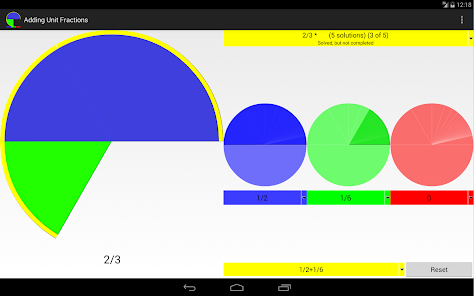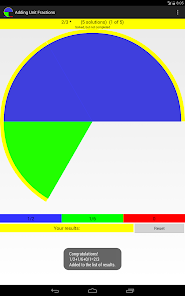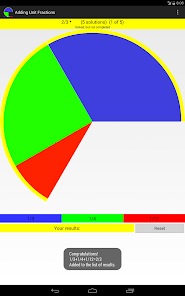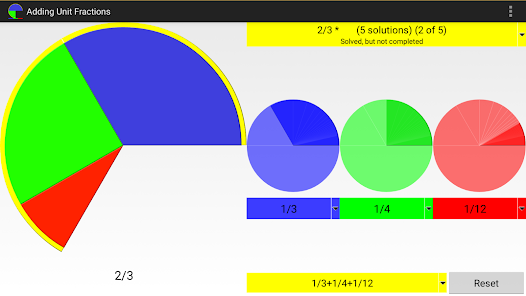The app proposes 21 challenges to overcome.
Obtaining the proper fractions listed at the top of the application, adding two or three unit fractions.

Each proposed proper fraction has a variable number of solutions.
And different levels of difficulty

You can not repeat unit fractions with the same value.
In the app you'll find a button to delete all the solutions found in the current problem, and to start from scratch.
The littlest unit fraction used in this app is 1/28.

The program is designed to show the usefulness of the subtraction of fractions in solving such problems.

From www.nummolt.com
This is an evolution of the "Old Egyptian Fractions" made in collaboration with www.mathcats.com

Hint:
In the Rhind Mathematical Papyrus (RMP) in 1650 BC the scribe Ahmes copied the now-lost test from the reign of the king Amenemamhat III .
The first part of the papyrus is taken up by the 2/n table. The fractions 2/n for odd n ranging from 3 to 101 are expressed as sums of unit fractions.
In this app you can build some of the Ahmes decompositions ( 2/3 , 2/5, 2/7, 2/9 ) and the discarded ones by him also.
The app allow to decompose also: 3/4 , 3/5 , 4/5 , 5/6 , 3/7 , 4/7 , 5/7 , 3/8 , 5/8 , 7/8 , 4/9 , 5/9 , 7/9 , 8/9 , 3/10 , 7/10 , 9/10.
You can use the knowledge acquired solving the 2/n decompositions to solve the rest of the problems.

The app "Proper Fractions" (same developer) is the right tool that helps to solve 'adding unit fractions'

Merlot reference of this app:
https://www.merlot.org/merlot/viewMaterial.htm?id=917779
Courses:
Math 1, 2 and 3: Fractions
Math 4: Writing fractions, Equivalent fractions, Comparing and ordering, Simplifying fractions, Adding fractions, Subtracting fractions
Math 5, 6 and 7: Writing fractions, Equivalent fractions, Comparing & ordering, Simplifying fractions, Adding fractions, Subtracting fractions, Multiplying fractions, Dividing fractions

From: nummolt.com
Nummolt apps:
"Mathematics is the toughest toy. However mischievous a child may be, will never be able to break them".
Updated on
Nov 16, 2023

## Data safety

Safety starts with understanding how developers collect and share your data. Data privacy and security practices may vary based on your use, region, and age. The developer provided this information and may update it over time.No data shared with third parties